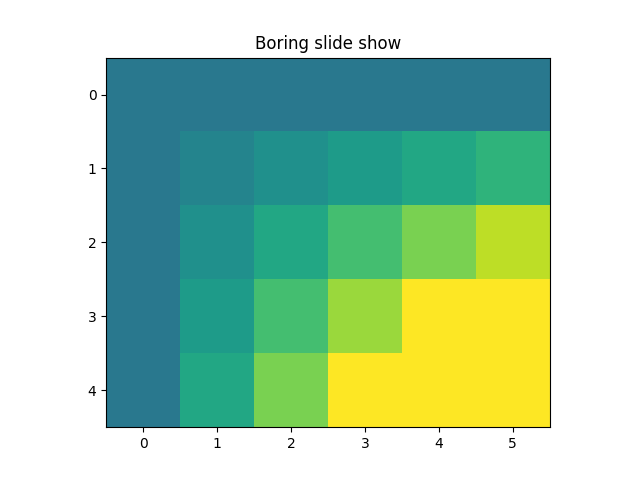Travis-CI:# Animation Demo¶

Pyplot animation example.

The method shown here is only for very simple, low-performance use. For more demanding applications, look at the animation module and the examples that use it.Out:

```step 0
step 1
step 2
step 3
step 4
```

```import matplotlib.pyplot as plt
import numpy as np

x = np.arange(6)
y = np.arange(5)
z = x * y[:, np.newaxis]

for i in range(5):
if i == 0:
p = plt.imshow(z)
fig = plt.gcf()
plt.clim()   # clamp the color limits
plt.title("Boring slide show")
else:
z = z + 2
p.set_data(z)

print("step", i)
plt.pause(0.5)
```

Total running time of the script: ( 0 minutes 2.652 seconds)

Gallery generated by Sphinx-Gallery